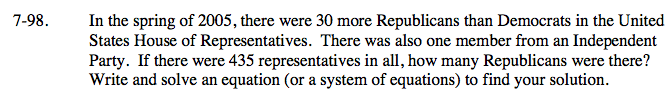### Home > AC > Chapter 7 > Lesson 7.3.2 > Problem7-98

7-98.

In the spring of 2005, there were 30 more Republicans than Democrats in the United States House of Representatives. There was also one member from an Independent Party. If there were 435 representatives in all, how many Republicans were there? Write and solve an equation (or a system of equations) to find your solution. Homework Help ✎Write an equation for the problem. x + (x − 30) + 1 = 435

x = the number of Republicans

Add like terms and solve for x to find the number of Republicans.
2x − 29 = 435
2x = 464
x = 232

There were 232 Republicans in the United States House of Representatives.